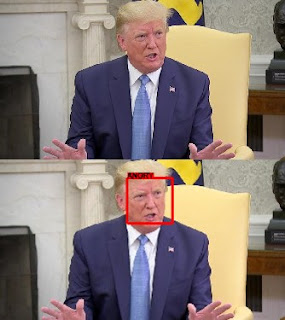### Emotion prediction using Deep Learning

According to me one of the most interesting field in Artificial intelligence is computer vision. It really interesting because through computer vision we can use images/videos to extract information. So, in this post, I am going to explore computer vision. We are going to build a facial expression recognition system using Deep Learning.### Tools and Data used

So the tools I have used are:

• Python
• Tensorflow and Keras
• OpenCV (cv2)
• Numpy and Pandas

The data set used for this is Fer2013. You can download the data using this link https://www.kaggle.com/deadskull7/fer2013. You can find the whole project here (https://github.com/abhimanyu1996/Emotion-Recognition-Fer2013)

### Implementation

Firstly we will prepare the data, then we will create a Convolutional Neural Network. After that, we will train our Neural network. Then finally we will use our neural network to detect the emotions of people in images and videos.

So let us start by reading the CSV file and defining the emotions

``````data = pd.read_csv("input/fer2013.csv")
data.pixels = data.pixels.str.split()
img_size = 48
emotion_to_str = {0:"ANGRY", 1:"DISGUST", 2:"FEAR", 3:"HAPPY", 4:"SAD", 5:"SURPRISE", 6:"NEUTRAL"}``````Now let's prepare the data so that we can fit it in our model.

``````def process_dataframe_images(images):
images = np.array(list(images), dtype=np.int)
images = images.reshape(-1,img_size, img_size,1)
return images

def images_to_data(images):
images = images/255.
return images

def data_to_images(imgdata):
imgdata = np.int(255.*imgdata)
imgdata[imgdata > 255] = 255
imgdata[imgdata < 0] = 0
return imgdata

training_data = data[data.Usage == "Training"][["emotion", "pixels"]]
print("Training Dataset:", len(training_data))
training_targets = training_data.emotion.values
training_images = process_dataframe_images(training_data.pixels.values)
training_images = images_to_data(training_images)

PrivateTest_data = data[data.Usage == "PrivateTest"][["emotion", "pixels"]]
print("PrivateTest Dataset:", len(PrivateTest_data))
PrivateTest_targets = PrivateTest_data.emotion.values
PrivateTest_images = process_dataframe_images(PrivateTest_data.pixels.values)
PrivateTest_images = images_to_data(PrivateTest_images)

PublicTest_data = data[data.Usage == "PublicTest"][["emotion", "pixels"]]
print("PublicTest Dataset:", len(PublicTest_data))
PublicTest_targets = PublicTest_data.emotion.values
PublicTest_images = process_dataframe_images(PublicTest_data.pixels.values)
PublicTest_images = images_to_data(PublicTest_images)``````

#### Create and Fit Model

Now that we have processed our data and converted it into images. Let's create a model. I am using a model with convolution layers.

``````def getModel():
model = tf.keras.Sequential()

input_shape=(img_size, img_size, 1), data_format='channels_last', kernel_regularizer=l2(0.01)))

return model``````

So we have created a model with 8 Convolutional layers. Now, let's fit our model.

``````K.clear_session()
num_labels = len(emotion_to_str)
num_features = 32
epochs = 100
batch_size = 128
model = getModel()
model.summary()
model.compile(loss="sparse_categorical_crossentropy",
metrics=['accuracy'])

filepath="weights/weights.best.hdf5"
checkpoint = ModelCheckpoint(filepath, monitor='val_acc', verbose=1, save_best_only=True, mode='max')
callbacks_list = [checkpoint]

model.fit(training_images, training_targets,
epochs=epochs, batch_size=batch_size,
validation_data=(PrivateTest_images, PrivateTest_targets),
shuffle=True, callbacks=callbacks_list)``````

So we created a checkpoint to save the best model. Now the best model accuracy I got was

```Public Test:
Loss: 1.130327940983638
Accuracy: 0.5826135
Private Test:
Loss: 1.098867057624651
Accuracy: 0.5842853```

Though the result is not good enough, but it is good enough for this project. You can try to tweak the model, adding some layers to improve the result. Let me know if you guys receive better results than these.

#### Predict Images and Videos

Now that we have fitted the model, Let us now use the model to predict the emotions of people in images. So the problem here is that the images we used for our model contains only the faces. So we have to take out the faces from the images first. We can use Haar cascades to get the faces. Now that we have the faces, we can process these faces and get the emotions out of these faces. Now let's code this.

``````face_cascade = cv2.CascadeClassifier('content/haar.xml')

def detect_image_emotion(img):
grayImg = cv2.cvtColor(img, cv2.COLOR_BGR2GRAY)
imgcopy = img.copy()

for (x,y,w,h) in faces:

facearray_gray = grayImg[y:y+h, x:x+w]

width_original = facearray_gray.shape
height_original = facearray_gray.shape

faceimg_gray = cv2.resize(facearray_gray, (img_size, img_size))
faceimg_gray = faceimg_gray/255.

faceimg_model = np.reshape(faceimg_gray, (1,img_size,img_size,1))
keypoints = model.predict(faceimg_model)

rectangle_bgr = (0, 0, 255)
font                   = cv2.FONT_HERSHEY_SIMPLEX
bottomLeftCornerOfText = (x,y)
fontScale              = 0.7
fontColor              = (0,0,0)
thickness               = 2

text = emotion_to_str[np.argmax(keypoints)]
(text_width, text_height) = cv2.getTextSize(text, font, fontScale=fontScale, thickness=thickness)
text_offset_x = x
text_offset_y = y
box_coords = ((text_offset_x, text_offset_y),
(text_offset_x + text_width, text_offset_y - text_height ))

cv2.rectangle(imgcopy,(x,y),(x+h,y+w),rectangle_bgr,5)
cv2.rectangle(imgcopy, box_coords, box_coords, rectangle_bgr, cv2.FILLED)
cv2.putText(imgcopy, text, (text_offset_x, text_offset_y), font,
fontScale=fontScale, color=fontColor, thickness=thickness)

return imgcopy``````

This function will return the processed image with a rectangle for each face with its emotion at the top. Now, let's use this function for emotion detection for an image.

``````img = cv2.imread('content/TestImage.jpg')
processed_img = detect_image_emotion(img)
plt.imshow(processed_img)``````
````

You can input your image as well just change the path for image. We can use the same function detect_image_emotion to process the images as well. Let us code that as well.

``````cap = cv2.VideoCapture('content/TestVideo3.mp4')
video_shape = (int(cap.get(3)), int(cap.get(4)))

fourcc = cv2.VideoWriter_fourcc(*'mp4v')
out = cv2.VideoWriter('content/output.mp4',fourcc, 20.0, video_shape, True)

while ret:
predict_image = detect_image_emotion(frame)
out.write(predict_image)
``cap = cv2.VideoCapture(0)``